Next: Afterword Up: Annotation Previous: Possible mechanism   Contents

# Remarks on some hypotheses

In this Appendix we shall touch upon some well-known hypotheses, which do not directly connected with the main part of the book. We begin with discussion of gravitation. The same dependence on distance for both gravitational and electromagnetic forces urges on an incorrect idea that there exists the single universal mechanism of action for these forces and gravitation could be explained by means of an electromagnetic field; however, it contradicts experiments (for example, it does not be found any shielding of gravitation). The gravitational force cannot be some force of Van der Waals' type, otherwise some long-range force, which weakly decreases with the distance, must exist (to obtain the squared dependence in the denominator, as in the Newton law), but it is absent. It is also incorrect an attempt to symmetrize gravitation by means of introducing "mass charge" with different signs. Gravitation manifests itself only as the attracting force. In addition to the banal question "where hides antigravitation?", there exists a trivial refutation of "charge" approach. Let us consider a large body, for example, the Earth. Let it be "charged", for example, by "positive mass charge", and attracted bodies be "charged" by "negative mass charge". Consider the opposite process (Fig. C.1).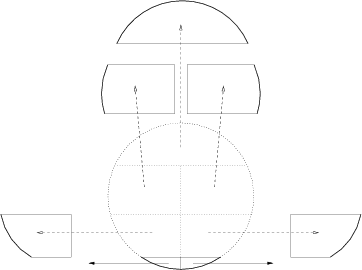We shall tear off big fragments from the Earth and take away far in space. It is well-known, that fragments which are heaved from the Earth, do not fly away by themselves in space, but fall back on the Earth. Therefore, positive "mass charge" must "flow down" on the remaining Earth after each such process. In this case its quantity will increase (to conserve the total "charge"). The last remaining fragmentwill attract bodies with a force that is large than one from the usual existing Earth. This is contradicts the proportionality of the gravitational force to the quantity of matter. Besides, there exists other contradiction: if the last fragmenthad been tearing off strictly in half, then which of two halves would be positive and which be negative? Or, by tearing off in half, the parts will repelling each other and we will have antigravitation? (Although, the presence or absence of antigravitation could be not connected with the presence or absence of negative mass.) The incorrect attempt of geometrization of gravitation provokes attempts of geometrization of other fields, for example, electromagnetic one. Error of this idea is obvious: besides charged particles, there exist neutral ones which do not "feel" charges till they collide "head-on" with some particle. Therefore, in the same point of space one particle would demonstrate a curved space, whereas other particle would prove absence of the curvature. Generally speaking, all above considered methods of formal reduction of one unknown force to some other unknown force have shown little promise.

It can be more useful to generalize the Newton static theory of gravitation with using of Maxwell approach (see , for example). Besides, there exists one more well-known interesting model. Unfortunately, mechanistic models are being permanently incited us as "something low-grade". But this is incorrectly. Similar models are the unique ones which can be created; we can "touch" they "by hands" and test capacity for work. They can be understood by anybody (from a schoolboy to a famous scientist), and anyone can discuss they (contrary to models which are "completely proved among several scientist of a particular school of thought"). The model under consideration consists in the following. It is assumed that in the Universe very small neutral particles ("Lesagens"; the author - LeSage) fly in all directions uniformly and interchange momentums with bodies in elastic collisions. Two bodies cast shadows (or penumbra) to each other, and, as a result, they attract each other with the force that varies in inverse proportion to the square of the distance. But there exists one "but". Since protons and electrons are opaque for these hypothetical particles, so it will be observed the departure of the mass dependence of attractive force from the proportionality to the product of masses for bodies with large sizes (with radii of the order of thousands kilometers and more). Unfortunately, this cannot be confirmed or disproved in experiments for the present. There existed yet another objection: a temperature of the Lesage's gas must be very great, and the Universe must "burn", since a thermodynamical equilibrium must quickly be established. However, subsequent modifications of this theory came already into being: 1) new lesagens can permanently be absorbed by bodies (the latters are permanently "growing" therewith); 2) lesagens can be transformed into such particles, which can desert the body. Gravitation is not completely investigated even from the experimental viewpoint. For example, no precision measurements exist, which study the influence of the mutual motion and rotation of bodies on the attractive gravitational force acting between them. There exist hypotheses of gravitational influence on the inert mass (and, therefore, on inertial forces, which arise in a rotating whipping top, for example). There arises a question (as some manifestation of relativistic cliches inculcated us): relative what must the rotation be determined? There exists a practical method principally to verify an inertial system. Since we can define the variation of a state (an extension of a spring between two rotating balls, for example) relative some other previous state only, it can be affirmed that the extension (due to an action of the centrifugal force) will be minimal for some frequency of rotation (naturally, considering the possible change in the direction of rotation). If this state of minimal extension is maintained independently on orientation of rotation axis, then we have some inertial system. The question, whether it will be the heliocentric system or other one, cannot be solved from pure theoretical considerations for our sole Universe (it is no sense to abstract theorize: it is practically impossible to remove almost all bodies from the Universe). It is obvious that inertial forces have the same mathematical form, and we can discuss a dependence of the inert mass itself on gravitation only. Probably, any detectable dependence of the inert mass on the direction of the resulting gravitational vector is impossible (alternatively, rotating liquids in the state of weightlessness could not be observed as ellipsoid of rotation, for example). Any noticeable dependence on the absolute value of the resulting gravitational vector is also improbable: in the opposite case calculations of motion of comets, asteroids and meteorites were differ from accepted data by exponents (for example, due to the law of conservation of linear momentum, the velocity of a body which were moving away from massive bodies, such as the Earth, the Sun etc., would be increased, but it is not the case). At first, to discuss a dependence of the inert mass on the value of the total gravitational potential (for small variations in motion at great distances), it is necessary to define, from the all-physical and general-philosophical viewpoints, what meaning of the zero level of this potential, and what the method of its determination in our sole Universe (to make some quantitative evaluations). It seems reasonable to say that this dependence of the inert mass cannot also be appreciable (see the discussion on the Mach principle in the book). But, in the general case the problem can principally be solved by experiments only. A row of cosmological problems could be theoretically solved, if it was assumed a boundedness of the radius of gravitational interactions . But it is impossible yet to check this hypothesis, since the effect becomes remarkable for the great astronomical distances only. So, the theory of gravitation remained in the same state as it was left by Newton. This field of knowledge waits for serious investigators.

Now we will mention additional hypotheses that try to answer on the following question: "what is the matter of light by itself?" The postulate of corpuscular-wave dualism should not paralyze the human thought. It is impossible to manage without corpuscular properties. And since it is rather simple to imitate the wave properties with the help of particles (recall the real phenomena: sound in the air, sea waves, etc.), so at present it is also urgent Newton's opinion that "light is rather corpuscules than waves". But light can represent a pure wave, or it can be an intermediate something with a complex inner structure. This allows to construct different models of light (Fig. C.2).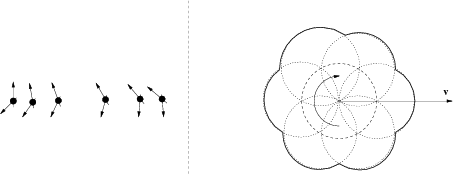Light can be described even by a longitudinal wave (despite the experiment on polarization) in the case of oriented properties of light particles. Or it can be represented as some likeness of a "rotating gear". In this case the electromagnetic wave influence on a medium or instrument can be associated with angular frequency of revolution of the "gear" and even can lead to the relationship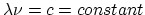. However, such a local (inside the instrument) speed of light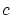can be absolutely not connected with the velocity of motion of the "gear" as a whole (with the velocity of passage of the given distance by light in space). Assuming the own rotation of photon and the classical law for velocity addition, the Doppler effect, which coincides with the relativistic one within the grasp of the present state of the art (correct to second order in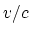), was obtained in . Some investigators have doubts even on the conventional Lebedev experiments (on the existence of the light pressure): firstly, some comets fly with tail forward to the Sun; secondly, evaluations show a too small effect, but a considerably greater value for the radiometrical effect. Unfortunately, the questions about the nature of light are also not solved both from theoretical and practical points of view. They also wait investigators.

The more large field relates the foundations of electrodynamics, but practically we do not touch it in the present book. Although achievements in this field for practical applications are really huge, nevertheless harmony in the conventional theory has not been feeling . Many pieces of the theory are seemed as artificially joined to each other. At least there exist many unsolved methodical problems here. If we will believe truth of the differential Maxwell equations, then, instead of the Lorentz force, some other "closing expression"  can strictly be obtained and some interesting solutions. Also we mention briefly the interesting idea of the new axiomatic approach to electrodynamics , attempts to revive Hertz's electrodynamics and to generalize Weber's force . Recall that original Weber's force was abandoned for the following reason: at some initial conditions it resulted in the self-acceleration of charges. The similar self-acceleration of charges under the action of the braking due to radiation was "discovered" in SRT also, but, for some strange reasons, SRT did not be rejected (a "double standard" is observed). At present the problem of self-acceleration (and other problem of the angular dependence of acceleration) has been rather successfully solving within the framework of Weber's force.

The hypotheses of the given Appendix were mentioned only to awake reader's interest in independent researches.Next: Afterword Up: Annotation Previous: Possible mechanism   Contents
Sergey N. Arteha